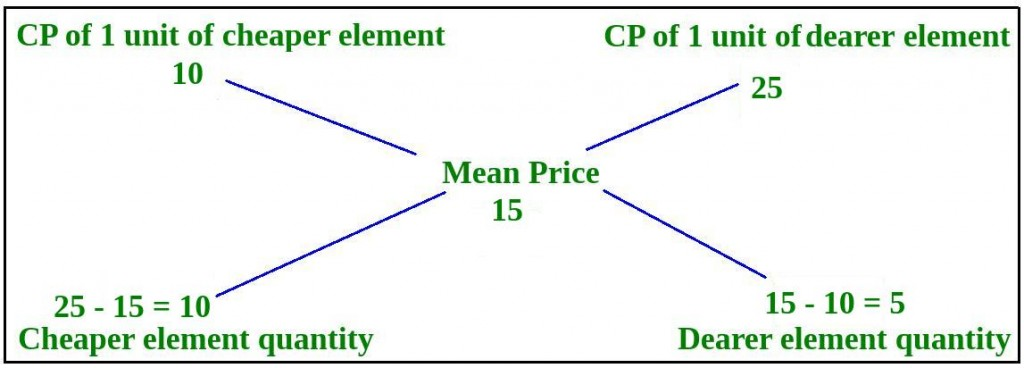Open in App
Not now

# Mixture and Alligation

• Difficulty Level : Medium
• Last Updated : 28 Jun, 2021

### Sample Problems

Question 1 : From a vessel of 20 liters pure milk, 1 liter is taken out and replaced with water, so as to keep the volume constant to 20 liters. This process is repeated 5 times. Find the percentage of pure milk left in the vessel after 5 replacements.
Solution : Here, we need to apply the formula P x [1 – (R / P)]n
P = Initial quantity of pure element = 20 liters
R = Quantity replaced every time = 1 liter
n = Number of replacements = 5
So, quantity of pure milk after 5 replacements = 20 x [1 – (1 / 20)]5
Quantity of pure milk after 5 replacements = 20 x (0.95)5 = 20 x 0.773780938 = 15.4756 liters
Therefore, percentage of pure milk left in the vessel after 5 replacements = (15.4756 / 20) x 100 = 77.378 %

Question 2 : A dishonest shopkeeper mixed cheaper quality of rice, priced at Rs. 10 / KG with good quality rice, priced at Rs. 25 / KG and sells the mixture at Rs. 15 / KG. Find the ratio in which he mixes the two qualities of rice.
Solution :Thus, ratio of quantities of cheaper and good quality rice = 10 : 5 = 2 : 1

### Problems on Mixture and Alligation | Set 2

Programs on Mixture and Alligation